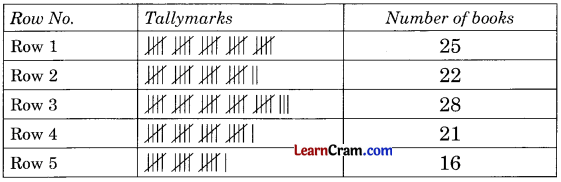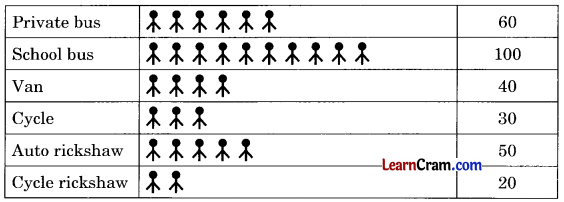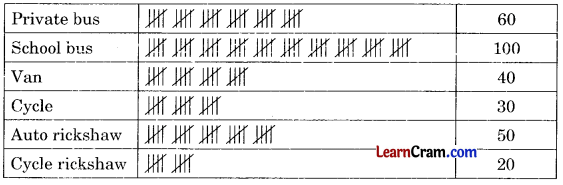# DAV Class 6 Maths Chapter 16 Worksheet 1 Solutions

The DAV Class 6 Maths Book Solutions and DAV Class 6 Maths Chapter 16 Worksheet 1 Solutions of Statistics offer comprehensive answers to textbook questions.

## DAV Class 6 Maths Ch 16 WS 1 Solutions

Question 1.
Below is given the sale of electric bulbs in a shop of different days of a week. Arrange the data in a table using tally marks.

 Monday 6 Tuesday 8 Wednesday 9 Thursday 11 Friday 7 Saturday 2Question 2.
The number of different varieties of toffees are given below. Arrange the data in a table using tally marks.

 Mango bites 12 Eclairs 16 Coffee -toffee 13 Lacto plus 18 Melody 11Question 3.
The number of books in each row of a cupboard is given below. Arrange the data in a table using tally marks

 Row 1 25 Row 2 22 Row 3 28 Row 4 21 Row 5 16### DAV Class 6 Maths Chapter 16 Worksheet 1 Notes

The following are the methods to represent the given data:

• By pictograph
• By bar graph
• By tally marks
• By pie chart

Example 1:
Following table represents the information about the school-going students. Here symbol indicates = 10 students.Look at the above table, find out the following information.
(a) How many students use cycle rickshaw?
(b) How many students avail the school bus facility?
(c) If there are 500 students in the school, then how many students do not use any type of conveyance?
Solution:
(a) Only 20 students use cycle rickshaw.
(b) 100 students avail the school bus facility.
(c) Total number of students in the school = 500
Number of students who use conveyance = 300
∴ Number of students who do not use any type of conveyance = 500 – 300 = 200

Example 2:
Use tally marks to represent the information given in Example 1.
Here symbol indicates = 10 students.
Solution: